# Power Flow Transfer Equations

## Power Flow Transfer Equations:

Power Flow Transfer Equations – The flow of active and reactive power in a synchronous link will now be studied. The approach will be analytical and armature resistance will be considered for generality of results.

Figure 8.42 shows the schematic diagram of a synchronous generator wherein E̅f leads V̅t by angle δ. The synchronous impedance isas shown by the impedance triangle of Fig. 8.38 whereinThe armature current in Fig. 8.42 can be expressed asThe complex power output isSubstituting for /a from Eq. (8.49) in Eq. (8.50),Equating the real and imaginary parts of Eq. (8.51), the following expressions for real and reactive power output are obtained asThe net mechanical power input to the machine is given byIt is convenient to express the above results in terms of angle a defined in the impedance triangle of Fig. 8.43 (Eq. 8.48b). Equations (8.52a), (8.52b) and (8.53) then modify as below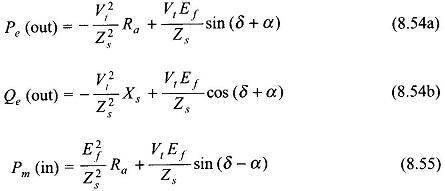The real electrical power output, Pe, as per Eq. (8.54a) is plotted in Fig. 8.44 from which it is observed that its maximum value is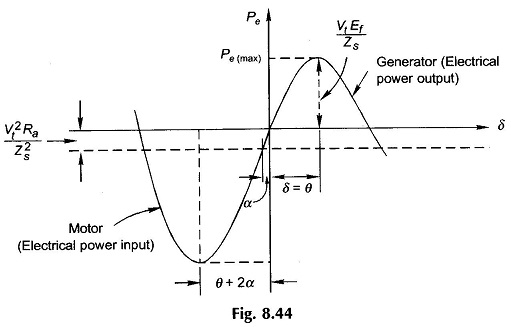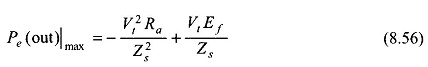occurring at δ= θ, which defines the limit of steady-state stability. The machine will fall out of step for angle δ> θ. Of course, θ will be 90° if resistance is negligible in which case the stability limit will be at δ= 90° as already explained in Sec. 8.8

It follows from Eq. (8.55) that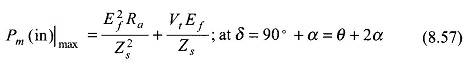Since the angle δ in Eq. (8.57) is more than θ, the maximum mechanical power input (net) operation for a generator lies in the unstable region.

Equations (8.54a), (8.54b), (8.55) and (8.56) simplify as below when armature resistance is neglected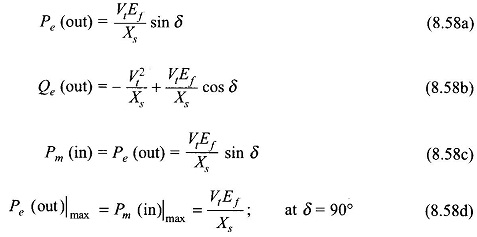Figure 8.45 shows the operation of the synchronous machine as a motor*. Here the angle δ by which Ef lags Vt is defined as positive. Also the direction of power flow is now into the machine while the mechanical power flows out at the machine.It then follows from Eqs (8.54a), (8.54b) and (8.55) by changing the sign of S that for motoring operation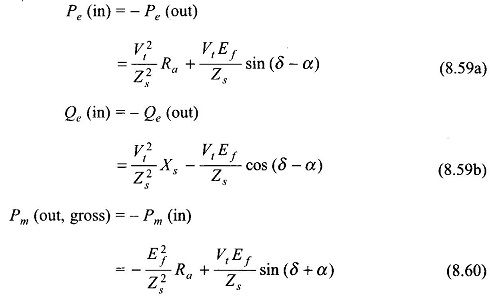The maximum mechanical power output from Eq. (8.60) is given by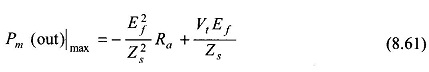It occurs at δ = θ which defines the limit of steady-state stability. It is easily seen from Eq. (8.59a) that the maximum electrical power input occurs at δ = θ + 2α which lies outside the stability limit. The reader should compare these results with that of the generator.

For the case of negligible resistanceFor unity pf operation Qe= 0 in Eq. (8.62b) which occurs when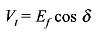This result is easily verified from Fig. 8.32(b) for the upf phasor diagram.